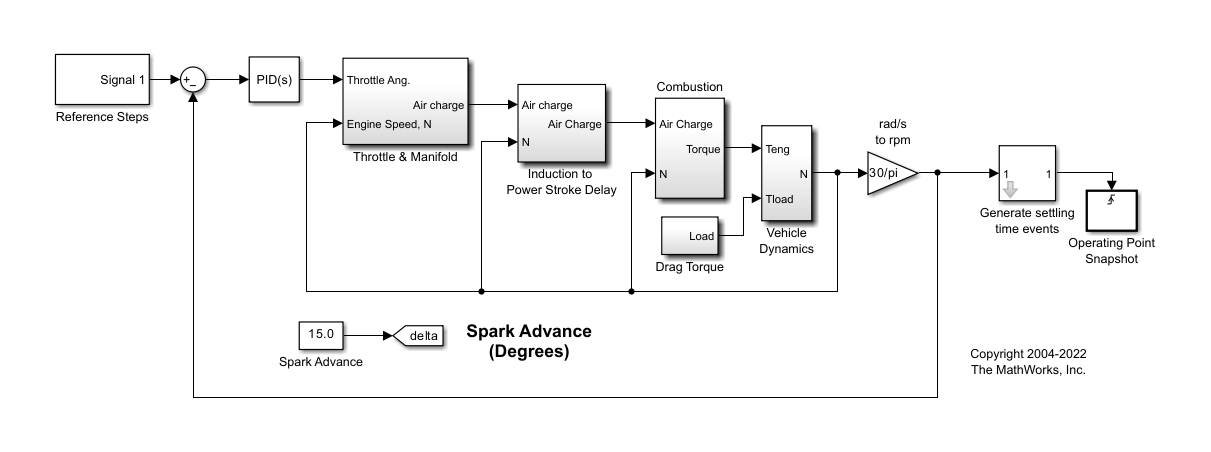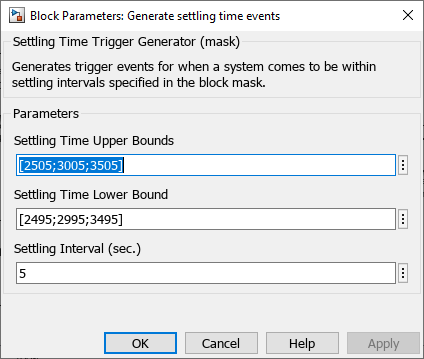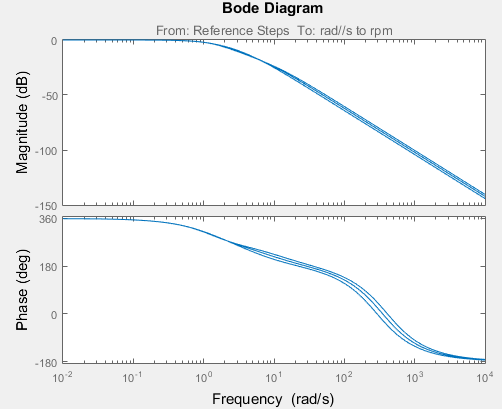Documentation

Linearize at Triggered Simulation Events

This example shows how to use the Linear Analysis Tool to linearize a model at specific events in time. Linearization events can be trigger-based events or function-call events. Specifically, the model is linearized at the steady-state operating points 2500, 3000, and 3500 rpm.

1. Open Simulink® model.

sys = 'scdspeedtrigger';
open_system(sys)To help identify when the system is at steady state, the Generate settling time events block generates settling events. This block sends rising edge trigger signals to the Operating Point Snapshot block when the engine speed settles near 2500, 3000, and 3500 rpm for a minimum of five seconds.The model already includes a Trigger-Based Operating Point Snapshot block from the Simulink Control Design™ library. This block linearizes the model when it receives rising edge trigger signals from the Generate settling time events block.

2. Compute the steady-state operating point at 60 time units.

op = findop(sys,60);

This command simulates the model for 60 time units, and extracts the operating points at each simulation event that occurs during this time interval.

3. Define the portion of the model to linearize.

io(1) = linio('scdspeedtrigger/Reference Steps',1,'input');
io(2) = linio('scdspeedtrigger/rad//s to rpm',1,'output');
4. Linearize the model.

linsys = linearize(sys,op(1:3),io);
5. Compare linearized models at 2500, 3000, and 3500 rpm using Bode plots of the closed-loop transfer functions.

bode(linsys);